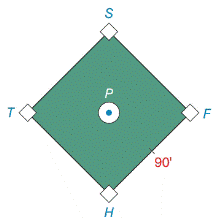Chapter 11.4, Problem 38EElementary Geometry For College St...

7th Edition
Alexander + 2 others
ISBN: 9781337614085

Solutions

Chapter
SectionElementary Geometry For College St...

7th Edition
Alexander + 2 others
ISBN: 9781337614085
Textbook Problem

On a baseball diamond (a square), the bases are 90  ft apart. Use the Law of Sines to find the distance from home plate to second base.Exercises 38 – 39

To determine

To find:

The distance from home place to second base of a baseball diamond

Explanation

Formula:

The Law of Sines,

sinαa=sinβb=sinγc

Where α, β, and γ is the acute angle of the triangle, and a, b, and c is the sides of the triangle.

Calculation:

Given,

The baseball diamond (square)

Let R be the home place S is the second base of the baseball diamond (square).

Here, length of bases are 90 ft.

Draw a line with the two points R and S

Then, RS¯ spilt the square as a two isosceles triangles whose length x.

By the isosceles triangle RFS,

F=90.

For any triangle

F+R+S=180

90+R+S=180

Since, R=S

90+R+R=180

90+2R=180

Add (90) on both sides,

90+2R90=18090

2R=90

Divide by 2 on both sides,

2R

Still sussing out bartleby?

Check out a sample textbook solution.

See a sample solution

The Solution to Your Study Problems

Bartleby provides explanations to thousands of textbook problems written by our experts, many with advanced degrees!

Get Started